Anzeige

# Project monitoring lecture 1

26. Dec 2019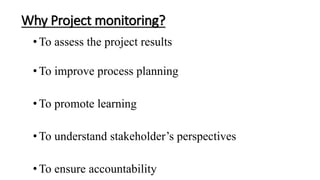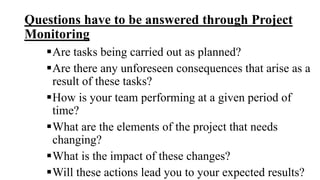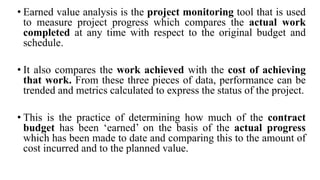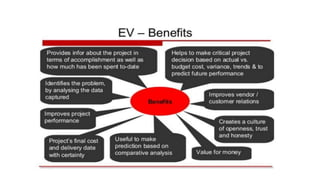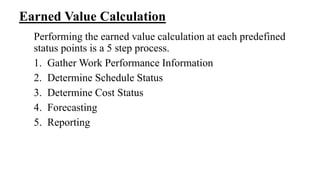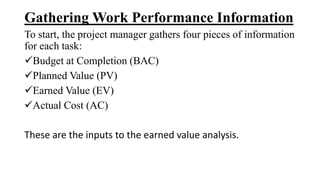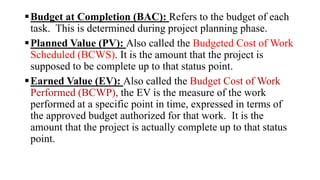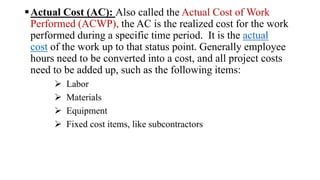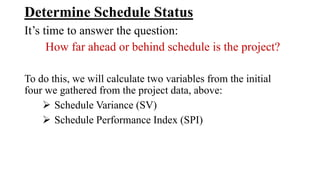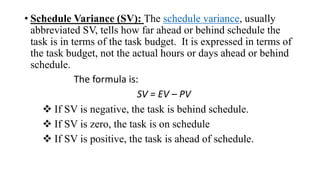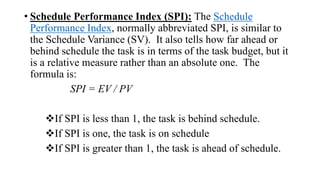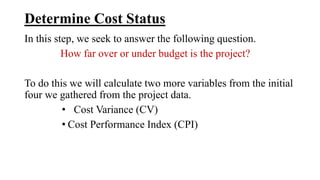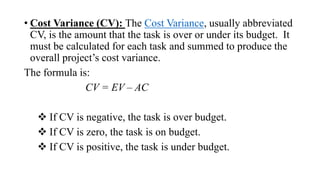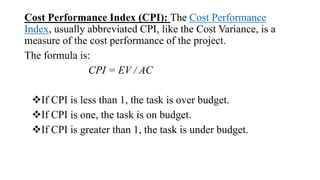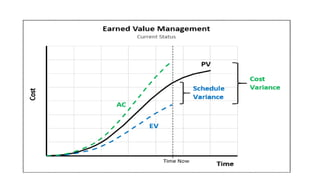1 von 18
Anzeige

### Project monitoring lecture 1

1. PROJECT MONITORING SHOWAIB AHMED CHOWDHURY Lecturer Department of Building Engineering & Construction Management Rajshahi University of Engineering & Technology(RUET)
2. Project Monitoring refers to the process of keeping track of all project-related metrics including team performance and task duration, identifying potential problems and taking corrective actions necessary to ensure that the project is within scope, on budget and meets the specified deadlines. It clarifies the objectives of the project, links the activities to the objectives, sets the target, reports the progress to the management and keeps the management aware of the problems which crop up during the implementation of the project.
3. Why Project monitoring? • To assess the project results •To improve process planning • To promote learning • To understand stakeholder’s perspectives • To ensure accountability
4. Questions have to be answered through Project Monitoring Are tasks being carried out as planned? Are there any unforeseen consequences that arise as a result of these tasks? How is your team performing at a given period of time? What are the elements of the project that needs changing? What is the impact of these changes? Will these actions lead you to your expected results?
5. EARNED VALUE ANALYSIS
6. • Earned value analysis is the project monitoring tool that is used to measure project progress which compares the actual work completed at any time with respect to the original budget and schedule. • It also compares the work achieved with the cost of achieving that work. From these three pieces of data, performance can be trended and metrics calculated to express the status of the project. • This is the practice of determining how much of the contract budget has been ‘earned’ on the basis of the actual progress which has been made to date and comparing this to the amount of cost incurred and to the planned value.
7. Earned Value Calculation Performing the earned value calculation at each predefined status points is a 5 step process. 1. Gather Work Performance Information 2. Determine Schedule Status 3. Determine Cost Status 4. Forecasting 5. Reporting
8. Gathering Work Performance Information To start, the project manager gathers four pieces of information for each task: Budget at Completion (BAC) Planned Value (PV) Earned Value (EV) Actual Cost (AC) These are the inputs to the earned value analysis.
9. Budget at Completion (BAC): Refers to the budget of each task. This is determined during project planning phase. Planned Value (PV): Also called the Budgeted Cost of Work Scheduled (BCWS). It is the amount that the project is supposed to be complete up to that status point. Earned Value (EV): Also called the Budget Cost of Work Performed (BCWP), the EV is the measure of the work performed at a specific point in time, expressed in terms of the approved budget authorized for that work. It is the amount that the project is actually complete up to that status point.
10. Actual Cost (AC): Also called the Actual Cost of Work Performed (ACWP), the AC is the realized cost for the work performed during a specific time period. It is the actual cost of the work up to that status point. Generally employee hours need to be converted into a cost, and all project costs need to be added up, such as the following items:  Labor  Materials  Equipment  Fixed cost items, like subcontractors
11. Determine Schedule Status It’s time to answer the question: How far ahead or behind schedule is the project? To do this, we will calculate two variables from the initial four we gathered from the project data, above:  Schedule Variance (SV)  Schedule Performance Index (SPI)
12. • Schedule Variance (SV): The schedule variance, usually abbreviated SV, tells how far ahead or behind schedule the task is in terms of the task budget. It is expressed in terms of the task budget, not the actual hours or days ahead or behind schedule. The formula is: SV = EV – PV  If SV is negative, the task is behind schedule.  If SV is zero, the task is on schedule  If SV is positive, the task is ahead of schedule.
13. • Schedule Performance Index (SPI): The Schedule Performance Index, normally abbreviated SPI, is similar to the Schedule Variance (SV). It also tells how far ahead or behind schedule the task is in terms of the task budget, but it is a relative measure rather than an absolute one. The formula is: SPI = EV / PV If SPI is less than 1, the task is behind schedule. If SPI is one, the task is on schedule If SPI is greater than 1, the task is ahead of schedule.
14. Determine Cost Status In this step, we seek to answer the following question. How far over or under budget is the project? To do this we will calculate two more variables from the initial four we gathered from the project data. • Cost Variance (CV) • Cost Performance Index (CPI)
15. • Cost Variance (CV): The Cost Variance, usually abbreviated CV, is the amount that the task is over or under its budget. It must be calculated for each task and summed to produce the overall project’s cost variance. The formula is: CV = EV – AC  If CV is negative, the task is over budget.  If CV is zero, the task is on budget.  If CV is positive, the task is under budget.
16. Cost Performance Index (CPI): The Cost Performance Index, usually abbreviated CPI, like the Cost Variance, is a measure of the cost performance of the project. The formula is: CPI = EV / AC If CPI is less than 1, the task is over budget. If CPI is one, the task is on budget. If CPI is greater than 1, the task is under budget.
Anzeige